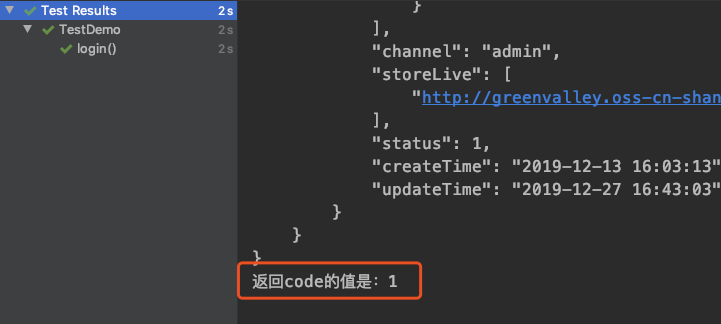# 利器 | Java 接口自动化测试首选方案：REST Assured 实践 (一)REST Assured 官方的 README 第一句话对进行了一个优点的概述，总的意思表达的就是简单好用。那么 REST Assured 有哪些优点，又该如何使用呢？

• 开源
• 简约的接口测试 DSL
• 支持 xml json 的结构化解析
• 支持 xpath jsonpath gpath 等多种解析方式
• 对 spring 的支持比较全面

``````<dependency>
<groupId>io.rest-assured</groupId>
<artifactId>rest-assured</artifactId>
<version>4.0.0</version>
<scope>test</scope>
</dependency>

``````

``````//使用参数
given().
param("key1", "value1").
param("key2", "value2").
when().
post("/somewhere").
then().
body(containsString("OK"))

//使用X-Path (XML only)
given().
params("firstName", "John", "lastName", "Doe").
when().
post("/greetMe").
then().
body(hasXPath("/greeting/firstName[text()='John']"))

``````

``````{
"scope": "server",
"userType": 1,
}

``````

``````Headers:    Authorization=Basic c3lzdGVtxxxRlbQ==
Host=47.103.xxx.133
Accept=*/*
Content-Type=application/json; charset=ISO-8859-1

``````

• param

• 通常我们都会使用 given().param 方法来传参，REST Assured 会根据 HTTP 方法自动尝试确定哪种参数类型（即查询或表单参数），如果是 GET，则查询参数将自动使用，如果使用 POST，则将使用表单参数；

• queryParam 和 formParam

• 有时候在 PUT 或 POST 请求中，需要区分查询参数和表单参数时，就需要使用queryParam 和 formParam 方法了，具体写法如下：

``````given().
formParam("formParamName", "value1").
queryParam("queryParamName", "value2").
when().
post("/something")

``````
• pathParam
使用given时指定请求路径的参数，这个方法很少用到，或者说我本人几乎没用到过(可能我的修行还不够，踩坑还太少~)；具体写法如下：
``````given().
pathParam("OAuth", "oauth").
pathParam("accessToken", "token").
when().
post("/auth/{OAuth}/{accessToken}").
then().
..

``````
``````given()

``````
``````given()

``````
``````given()

``````
• contentType
经常还会设置contentType，最常见的就是application/json了，写法如下：
``````given().contentType("application/json"). ..
//或者
given().contentType(ContentType.JSON). ..

``````
• body
在POST, PUT 或 DELETE请求中，我们经常还需要带上请求体body，写法如下：
``````given().body("{\n" +
"\t\"scope\": \"server\",\n" +
"\t\"userType\": 1,\n" +
"}")

``````

``````given().request().body("{\n" +
"\t\"scope\": \"server\",\n" +
"\t\"userType\": 1,\n" +
"}")

``````
• 没有参数
如果我们没有参数需要传递，也可以省略掉given()：
``````get("/lotto").then().assertThat().body("lotto.lottoId", equalTo(5));

``````
• proxy
有时候我们需要进行接口的调试，抓包是最常用的一种方式，rest-assured 提供了 proxy 方法，可以设置代理，写法如下：
``````given().proxy("127.0.0.1",8888). ..

``````

• when主要用来触发请求，在when后面接着请求URL：
``````given().when().post("http://47.103.xxx.133/auth/oauth/token"). ..

``````
• 前面在 given 中我们设置了很多请求参数，在 when 中也可以设置，只不过要注意的是在请求之前设置；这也比较好理解，如果再请求之后的话，参数都设置怎么发请求呢？
``````  given()
.when()
.contentType(ContentType.JSON)
.request().body("{\n" +
"\t\"scope\": \"server\",\n" +
"\t\"userType\": 1,\n" +
"}")
.post("http://47.xxx.xxx.133/auth/oauth/token")
. ..

``````
• 断言-then().body()
then().body() 可以对响应结果进行断言，在 body 中写入断言：
``````.. post("http://47.xxx.xxx.133/auth/oauth/token")
.then().statusCode(200).body("code",equalTo(1));

``````

• 获取响应-then().extract().body().path(“code”)
我们可以在 then 后面利用 .extract().body() 来获取我们想要 body 的返回值，它们也可以直接接在断言后面，写法如下：
``````.. .then()
.log().all().statusCode(200).body("code",equalTo(1))
.extract().body().path("code");

``````

``````body("{\n" +
"\t\"scope\": \"server\",\n" +
"\t\"userType\": 1,\n" +
"}")

``````

``````HashMap map = new HashMap();
map.put("scope","server");
map.put("userType",1);
given()
.contentType(JSON)
.body(map). ..

``````

``````HashMap map = new HashMap();
map.put("scope","server");
map.put("userType",1);
Integer code =
given()
.contentType(JSON)
.body(map).
when()
.log().all().post("http://47.xxx.xxx.133/auth/oauth/token").
then()
.log().all().statusCode(200).body("code",equalTo(1))
.extract().body().path("code");
System.out.println("返回code的值是："+code);

``````4 个月由浅入深，强化集训，测试大咖通过 8+ 企业级项目实战演练，带你一站式掌握 BAT 一线测试开发工程师必备核心技能（对标阿里巴巴P6+，挑战年薪50W+）！学员直推 BAT 名企测试经理，普遍涨薪 50%+！